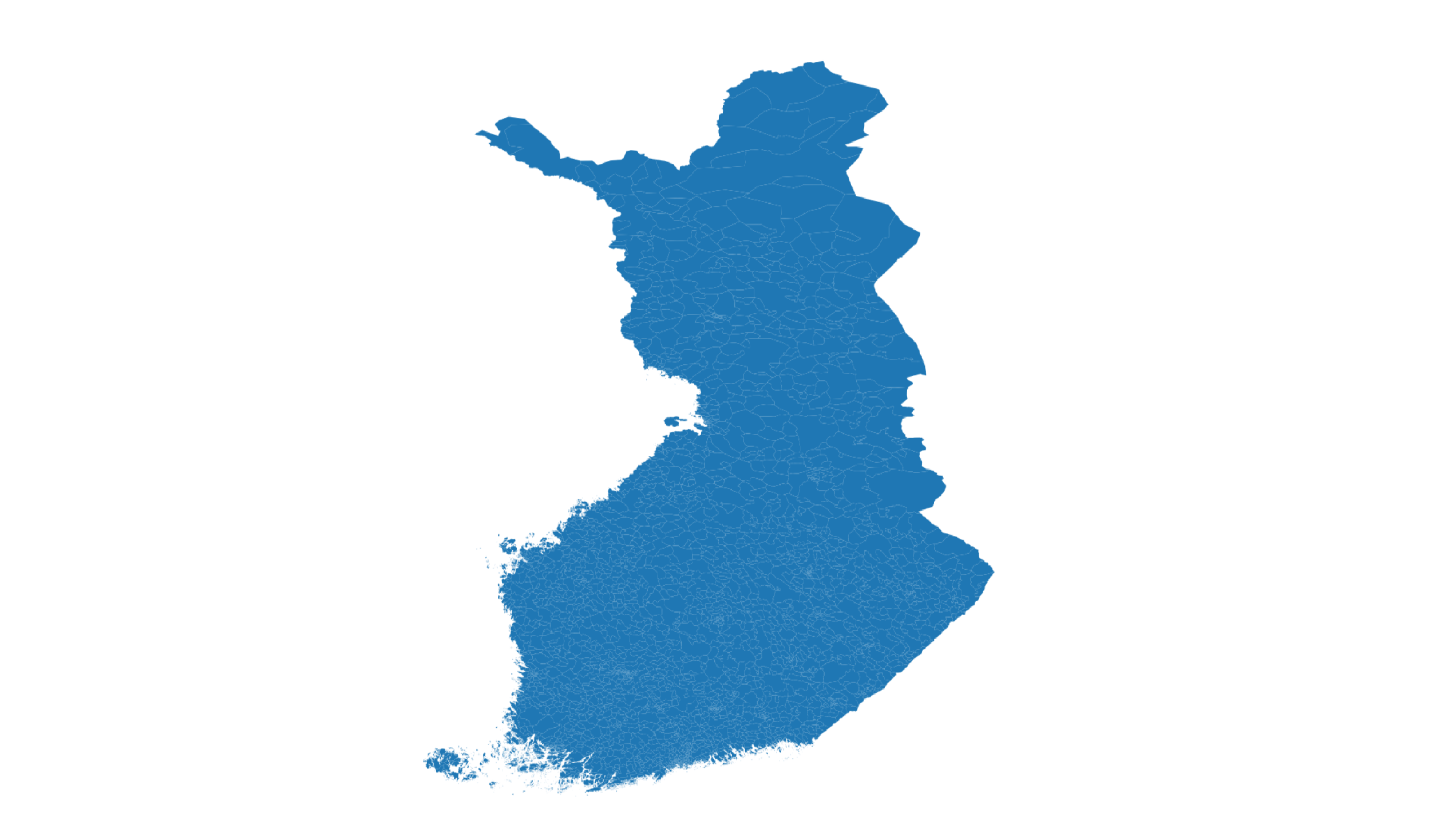Reading and visualizing Finland’s postal code data on a map in Python. Python has many great packages to work with geospatial such as `geopandas`.

## Python workspace for postal code visualization

The examples work best in `Jupyter notebook` environments.

If you don’t want to setup Python and the required libraries on your own, you can choose from these options free browser-based Python workspace.

Postal code data can be read into Python with `geopanads` library in `GetJSON` file format.

Here you can copy the Python code to get started with postal codes.

``````import matplotlib.pyplot as plt
import geopandas as gpd

input_file = "finland-postal-codes-sample.geojson"

#Derive a silly value from postal code to colorize the regions
gdf["fake_value"] = (gdf["postinumeroalue"].astype("int")-10000) / 30000

fig, ax = plt.subplots(figsize=(10,10))
#Set longitude limits to show in the map
plt.xlim(21.2, 24.5)
#Set latitude limits to show in the map
plt.ylim(59.8, 62)

#Use Finland map as a background
finland = world[(world.pop_est>0) & (world.name=="Finland")].copy()
finland.plot(ax=ax, color="lightgray", edgecolor='gray')

#Plot the postal code on top
gdf.plot(ax=ax, edgecolor="white", alpha=gdf.fake_value)
``````

A simple visualization of postal code data using Python’s `matplotlib` library looks like this:

With the full postal code dataset you can create similar map than below. You also need to leave out the plot’s `x` and `y` limits and possibly the background map.All postal code boundaries of Finland on a map in Python.

## Interactive map of zip code data in Python

You can create an interactive map in Python notebooks with the following script. The code requires the `folium` library to be installed.

``````import folium

m = folium.Map(
location=[61.1, 23.1],
tiles="cartodbpositron",
zoom_start=8,
)

input_file = "finland-postal-codes-sample.geojson"